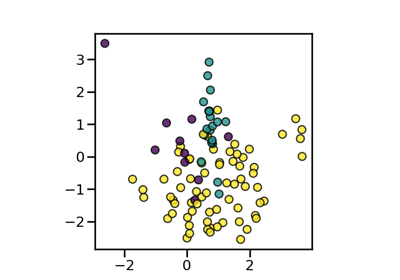# imblearn.combine.SMOTETomek¶

class imblearn.combine.SMOTETomek(*, sampling_strategy='auto', random_state=None, smote=None, tomek=None, n_jobs=None)[source]

Over-sampling using SMOTE and cleaning using Tomek links.

Combine over- and under-sampling using SMOTE and Tomek links.

Read more in the User Guide.

Parameters
sampling_strategyfloat, str, dict or callable, default=’auto’

Sampling information to resample the data set.

• When float, it corresponds to the desired ratio of the number of samples in the minority class over the number of samples in the majority class after resampling. Therefore, the ratio is expressed aswhereis the number of samples in the minority class after resampling andis the number of samples in the majority class.

Warning

float is only available for binary classification. An error is raised for multi-class classification.

• When str, specify the class targeted by the resampling. The number of samples in the different classes will be equalized. Possible choices are:

'minority': resample only the minority class;

'not minority': resample all classes but the minority class;

'not majority': resample all classes but the majority class;

'all': resample all classes;

'auto': equivalent to 'not majority'.

• When dict, the keys correspond to the targeted classes. The values correspond to the desired number of samples for each targeted class.

• When callable, function taking y and returns a dict. The keys correspond to the targeted classes. The values correspond to the desired number of samples for each class.

random_stateint, RandomState instance, default=None

Control the randomization of the algorithm.

• If int, random_state is the seed used by the random number generator;

• If RandomState instance, random_state is the random number generator;

• If None, the random number generator is the RandomState instance used by np.random.

smoteobject, default=None

The imblearn.over_sampling.SMOTE object to use. If not given, a imblearn.over_sampling.SMOTE object with default parameters will be given.

tomekobject, default=None

The imblearn.under_sampling.TomekLinks object to use. If not given, a imblearn.under_sampling.TomekLinks object with sampling strategy=’all’ will be given.

n_jobsint, default=None

Number of CPU cores used during the cross-validation loop. None means 1 unless in a joblib.parallel_backend context. -1 means using all processors. See Glossary for more details.

SMOTEENN

Over-sample using SMOTE followed by under-sampling using Edited Nearest Neighbours.

Notes

The methos is presented in .

Supports multi-class resampling. Refer to SMOTE and TomekLinks regarding the scheme which used.

References

1

G. Batista, B. Bazzan, M. Monard, “Balancing Training Data for Automated Annotation of Keywords: a Case Study,” In WOB, 10-18, 2003.

Examples

>>> from collections import Counter
>>> from sklearn.datasets import make_classification
>>> from imblearn.combine import SMOTETomek
>>> X, y = make_classification(n_classes=2, class_sep=2,
... weights=[0.1, 0.9], n_informative=3, n_redundant=1, flip_y=0,
... n_features=20, n_clusters_per_class=1, n_samples=1000, random_state=10)
>>> print('Original dataset shape %s' % Counter(y))
Original dataset shape Counter({1: 900, 0: 100})
>>> smt = SMOTETomek(random_state=42)
>>> X_res, y_res = smt.fit_resample(X, y)
>>> print('Resampled dataset shape %s' % Counter(y_res))
Resampled dataset shape Counter({0: 900, 1: 900})

__init__(self, *, sampling_strategy='auto', random_state=None, smote=None, tomek=None, n_jobs=None)[source]

Initialize self. See help(type(self)) for accurate signature.

## Examples using imblearn.combine.SMOTETomek¶